Foxtable(狐表)用户栏目专家坐堂 → 关于快递API中的ＭＤ5加密的问题

共有52人关注过本帖树形打印复制链接

# 主题：关于快递API中的ＭＤ5加密的问题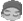ap9709130
1楼 | 信息 | 搜索 | 邮箱 | 主页 | UC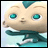加好友发短信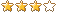老师      在快宝快递API 的开发文档中C#的函数如下:     public static string GetMd5(string md5str, int type)    {        if (type == 16)        {            MD5 algorithm = MD5.Create();            byte[] data = algorithm.ComputeHash(Encoding.UTF8.GetBytes(md5str));            string sh1 = "";            for (int i = 0; i < data.Length; i++)            {                sh1 += data[i].ToString("x2").ToUpperInvariant();            }            return sh1.Substring(8, 16).ToLower();        }        else if (type == 32)        {            MD5 algorithm = MD5.Create();            byte[] data = algorithm.ComputeHash(Encoding.UTF8.GetBytes(md5str));            string sh1 = "";            for (int i = 0; i < data.Length; i++)            {                sh1 += data[i].ToString("x2").ToUpperInvariant();            }            return sh1.ToLower();        }        return "";    }    我按论坛上的代码转换成VB.net 代码如下:     Public Shared Function GetMd5(ByVal md5str As String, ByVal type As Integer) As String    If type = 16 Then        Dim algorithm As MD5 = MD5.Create()        Dim data As Byte() = algorithm.ComputeHash(Encoding.UTF8.GetBytes(md5str))        Dim sh1 As String = ""        For i As Integer = 0 To data.Length - 1            sh1 += data(i).ToString("x2").ToUpperInvariant()        Next        Return sh1.Substring(8, 16).ToLower()    ElseIf type = 32 Then        Dim algorithm As MD5 = MD5.Create()        Dim data As Byte() = algorithm.ComputeHash(Encoding.UTF8.GetBytes(md5str))        Dim sh1 As String = ""        For i As Integer = 0 To data.Length - 1            sh1 += data(i).ToString("x2").ToUpperInvariant()        Next        Return sh1.ToLower()    End If    Return ""End Function但是    Dim algorithm As MD5 = MD5.Create() 这句会报错,说不存在 MD5 ,请问老师要怎么改 ?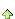2楼 | 信息 | 搜索 | 邮箱 | 主页 | UC加好友发短信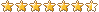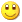Post By：2020/7/2 8:33:00 [只看该作者]

 写全命名空间Public Function GetMd5(ByVal md5str As String, ByVal Type As Integer) As String    If Type = 16 Then        Dim algorithm As System.Security.Cryptography.MD5 = System.Security.Cryptography.MD5.Create()        Dim data As Byte() = algorithm.ComputeHash(Encoding.UTF8.GetBytes(md5str))        Dim sh1 As String = ""        For i As Integer = 0 To data.Length - 1            sh1 += data(i).ToString("x2").ToUpperInvariant()        Next        Return sh1.Substring(8, 16).ToLower()    ElseIf Type = 32 Then        Dim algorithm As System.Security.Cryptography.MD5 = System.Security.Cryptography.MD5.Create()        Dim data As Byte() = algorithm.ComputeHash(Encoding.UTF8.GetBytes(md5str))        Dim sh1 As String = ""        For i As Integer = 0 To data.Length - 1            sh1 += data(i).ToString("x2").ToUpperInvariant()        Next        Return sh1.ToLower()    End If    Return ""End Function